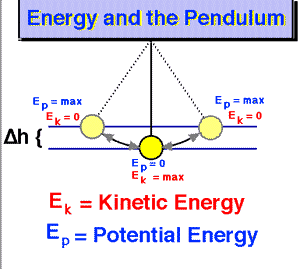Posted on

Energy – mechanical, potential, kineticenergy pendulum

Work is done upon an object whenever a force acts upon it to cause it to be displaced. Work involves a force acting upon an object to cause a displacement. In all instances in which work is done, there is an object that supplies the force in order to do the work.

An object that possesses some form of energy supplies the force to do the work. In the process of doing work, the object that is doing the work exchanges energy with the object upon which the work is done. When the work is done upon the object, that object gains energy. The energy acquired by the objects upon which work is done is known as mechanical energy.

Mechanical energy is the energy that is possessed by an object due to its motion or due to its position. Mechanical energy can be either kinetic energy (energy of motion) or potential energy (stored energy of position). Objects have mechanical energy if they are in motion and/or if they are at some position relative to a zero potential energy position.

For example, A moving baseball possesses mechanical energy due to both its high speed (kinetic energy) and its vertical position above the ground (gravitational potential energy).

Mechanical energy is often defined as the ability to do work. Any object that possesses mechanical energy – whether it is in the form of potential energy or kinetic energy – is able to do work. That is, its mechanical energy enables that object to apply a force to another object in order to cause it to be displaced.

```source and to read more: